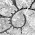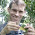## Tuesday, October 28, 2014

### Alternative implementations of fitContinuous

A colleague contacted me today about “alternative implementations” of geiger's `fitContinuous` function that he could use to check parameter estimation, and maybe to speed calculations as `fitContinuous` can be somewhat slow (although is evidently quite robust now) for some models.

Well, I don't know about speeding things up - but it is pretty straightforward to write a wrapper function that combines phytools `brownie.lite` single-rate model with geiger `rescale.phylo` to fit many of the models of `fitContinuous`. The following is a simple demo:

``````## load packages
library(phytools)
library(geiger)

## helper function to get the root node number
getRoot<-function(tree) Ntip(tree)+1

## here is our fitContinuous lite function
fitCont<-function(tree,x,model="BM",interval=NULL){
if(model!="BM"){
lk<-function(par,tree,x,model) brownie.lite(paintSubTree(rescale(tree,model,par),getRoot(tree),"1"),x)\$logL1
oFit<-optimize(lk,interval,tree=tree,x=x,model=model,maximum=TRUE)
cFit<-brownie.lite(paintSubTree(rescale(tree,model,oFit\$maximum),getRoot(tree),"1"),x)
obj<-list(par=oFit\$maximum,model=model,sig2=cFit\$sig2.single,a=cFit\$a.single,logLik=oFit\$objective)
} else {
fit<-brownie.lite(paintSubTree(tree,getRoot(tree),"1"),x)
obj<-list(model=model,sig2=fit\$sig2.single,a=fit\$a.single,logLik=fit\$logL1)
}
obj
}
``````

OK, now let's try it for simulated data:

``````## simulate tree
tree<-pbtree(n=100,scale=1)
## simulate data under model="BM"
x<-fastBM(tree)
fitContinuous(tree,x)
``````
``````## GEIGER-fitted comparative model of continuous data
##  fitted 'BM' model parameters:
##  sigsq = 0.940924
##  z0 = -0.186971
##
##  model summary:
##  log-likelihood = -58.714396
##  AIC = 121.428793
##  AICc = 121.552504
##  free parameters = 2
##
## Convergence diagnostics:
##  optimization iterations = 100
##  failed iterations = 0
##  frequency of best fit = 1.00
##
##  object summary:
##  'lik' -- likelihood function
##  'bnd' -- bounds for likelihood search
##  'res' -- optimization iteration summary
##  'opt' -- maximum likelihood parameter estimates
``````
``````fitCont(tree,x)
``````
``````## \$model
##  "BM"
##
## \$sig2
##  0.9409
##
## \$a
##  -0.187
##
## \$logLik
##  -58.71
``````
``````## simulate data under model="lambda"
x<-fastBM(rescale(tree,model="lambda",0.5))
fitContinuous(tree,x,model="lambda")
``````
``````## GEIGER-fitted comparative model of continuous data
##  fitted 'lambda' model parameters:
##  lambda = 0.729756
##  sigsq = 1.217453
##  z0 = 0.254493
##
##  model summary:
##  log-likelihood = -122.552971
##  AIC = 251.105941
##  AICc = 251.355941
##  free parameters = 3
##
## Convergence diagnostics:
##  optimization iterations = 100
##  failed iterations = 0
##  frequency of best fit = 0.32
##
##  object summary:
##  'lik' -- likelihood function
##  'bnd' -- bounds for likelihood search
##  'res' -- optimization iteration summary
##  'opt' -- maximum likelihood parameter estimates
``````
``````fitCont(tree,x,model="lambda",interval=c(0,1))
``````
``````## \$par
##  0.7298
##
## \$model
##  "lambda"
##
## \$sig2
##  1.217
##
## \$a
##  0.2545
##
## \$logLik
##  -122.6
``````
``````## simulate data under model="OU"
x<-fastBM(rescale(tree,model="OU",0.4))
fitContinuous(tree,x,model="OU")
``````
``````## GEIGER-fitted comparative model of continuous data
##  fitted 'OU' model parameters:
##  alpha = 0.536268
##  sigsq = 1.171551
##  z0 = 0.216378
##
##  model summary:
##  log-likelihood = -61.980067
##  AIC = 129.960133
##  AICc = 130.210133
##  free parameters = 3
##
## Convergence diagnostics:
##  optimization iterations = 100
##  failed iterations = 0
##  frequency of best fit = 0.60
##
##  object summary:
##  'lik' -- likelihood function
##  'bnd' -- bounds for likelihood search
##  'res' -- optimization iteration summary
##  'opt' -- maximum likelihood parameter estimates
``````
``````fitCont(tree,x,model="OU",interval=c(0,1))
``````
``````## \$par
##  0.5363
##
## \$model
##  "OU"
##
## \$sig2
##  1.172
##
## \$a
##  0.2164
##
## \$logLik
##  -61.98
``````

Running `system.time` with any of these examples (except for `model="BM"`) does not show any improvement of this ostensibly lighter implementation - but this is probably due to lots of unnecessary internals in `brownie.lite` for fitting a multi-rate model (which is not actually done here). Improving on this is thus pretty simple (but I will not do so here, nor now).

That's it.

1.I feel it is important to point out (in case any unaware paleo people find this post) that the branch-rescaling for OU is inappropriate for non-ultrametric trees, such as those containing fossil data, as commented recently by Graham in MEE.

http://onlinelibrary.wiley.com/doi/10.1111/2041-210X.12201/full

2.Thanks for the props Dave. But I think what Liam has done here is great as it really gets at the nuts and bolts of how these models work.

With regards the speed up in fitContinuous, altering the number of iterations will help. Right now the function tries to optimize from different starting points using different optimizers to ensure robustness, which really cranks up computation time. Ironically, the algorithm in geiger 2.0 is actually much faster that that in versions <1.98 but the sheer number of default iterations makes it unwieldy for large datasets. the number of iterations is set in the control list, e.g., :
fitContinuous(phy, data, model...... control=list(niter=4))

for most datasets, a modest number of iterations will be fine, in my experience

3.Oh, I agree, Graham -- Liam, this is a fantastic post and should give people a context for thinking about these models structurally, which is something I think all too few people actually, truly understand.

I just feel compelled to plaster warning signs on anything that could trip up unsuspecting paleo-people, perhaps because I deal with a lot of unsuspecting paleo-people as paleotree's maintainer.

4.Adding to what Graham states, if you are running GEIGER in a terminal environment (why wouldn't you?!?) it can take advantage of multiple processor cores (set by default to ncores=2, but you can let it use all available cores by specifying ncores=NULL), signficantly decreasing runtime. Using any sort of GUI (e.g. the CRAN GUI or RStudio) negates the possibility of using multiple cores. Don't do this.

1.In Windows this is not supported, so I generally don't think about it - but I probably should as lots of my code could be sped up by parallelization - and, by all accounts, in R it is easy. - Liam

5.Dear Liam, I am using fitcontinous from PCA scores of a skull (geometric morphometrics data) in order to compare different models (WB, BM, EB, Pagels delta and OU). In delta and OU models I receive a warning message that the parameter estimate appears at bounds. I read in some posts that one possible solution is to adjust the bounds of the parameters, I try but have not success, also I read that this could be related with low phylogenetical signal in the data (actually the lambda value was near to zero), in this latter scenario I think that there is not much left to do, I have not problem with only present the other models but surely one reviewer will request it

Note: due to the very large amount of spam, all comments are now automatically submitted for moderation.